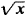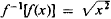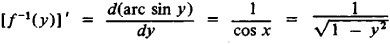# Inverse Function

(redirected from Invertible function)
Also found in: Dictionary, Thesaurus.
Related to Invertible function: Invertible matrix, Inverse functions

## inverse function

[′in‚vərs ′fənk·shən]
(mathematics)
An inverse function for a function ƒ is a function g whose domain is the range of ƒ and whose range is the domain of ƒ with the property that both ƒ composed with g and g composed with ƒ give the identity function.
McGraw-Hill Dictionary of Scientific & Technical Terms, 6E, Copyright © 2003 by The McGraw-Hill Companies, Inc.
The following article is from The Great Soviet Encyclopedia (1979). It might be outdated or ideologically biased.

## Inverse Function

a function that is the inverse of a given function. For example, if y = f(x) is a given function, then the variable x, considered as a function of the variable y, x = ø(y), is the inverse of the function y = f(x). For example, the inverse function of y = ax + b (α ≢ 0) is x = (y ø b)/a, the inverse function of y = ex is x = 1n y, and so forth. If x = ø(y) is the inverse function of y = f(x), then y = f(x) is the inverse function of x = ø(y).

The domain of definition of the given function is the range of values of the inverse function, and the range of the given function is the domain of the inverse function. The graphs of two mutually inverse functions y = f(x) and y = ø(x) (where the independent variable is designated by the same letter x), such as y = ax + b and y = (xb)/a or y = ex and y = 1n x, are symmetric with respect to the line y = x, which bisects the first and third quarters of the coordinate plane. The inverse of a single-valued function may be multiple-valued; compare, for example, the functions x2 and. A function y = f(x) has a single-valued inverse if and only if it takes on different values for different values of the independent variable. For a continuous function, this last condition can be satisfied only if the given function is monotonic (we have in mind real-valued functions of a real variable). The inverse of a continuous and monotonic function is single-valued, continuous, and monotonic.

If a given function is piecewise monotonic, then division of its domain of definition into regions of monotonicity yields single-valued branches of its inverse. For example, the interval –π/2 < x < π/2 serves as one of the regions of monotonicity of sin x. The branch of the inverse function Arc sin x that corresponds to this interval is the so-called principal branch arc sin x. The relations ø[f(x)] = x and f[ø(x)] = x hold for a pair of single-valued mutually inverse functions. The former holds for all values of x in the domain of definition of f(x), and the latter for all values of x in the domain of definition of ø(x); for example, e1nx = x(x > 0) and ln (ex) = x (— ∞ < x < ∞). The inverse of f(x) = y is sometimes designated as f1(y) = x, so that for a continuous and monotonie function f(x),

f–1[f(x)] = f[f–1f(x)] = x

In general, f–1[f(x)] is a multiple-valued function of x, one of whose values is x; for example, for f(x) = x2, x (≠ 0) is only one of the two values of(the other is – x); for f(x) = sin x, x is only one of an infinite set of values

f–1[f(x)] = Arc sin [sin x] = (–1)nx + nπ,

n = 0, ±1, ±2, …

If y = f(x) is continuous and monotonie in a neighborhood of x = x0 and has a nonzero derivative f’(x0) at x = x0, then f–1(y) is differentiable at y = y0, and

[f–1(y0)]’ = 1/f’(x0)

(the differentiation formula for an inverse function). Thus, for –π/2 < x < π/2,y = f(x) = sin x is continuous and monotonic and f’(x) = cos x ≠ 0. Then f–1(y) = arc sin y (–1 < y < 1) is differentiable, andHere the root is positive since cos x > 0 for –π/2 < x < π/2.